# SIL-VA COMMERCIALE S.R.L.

## Static characteristicsThe applied force acts in a direction perpendicular to the axis of the joint. The structure is characterized, in this case, by the maximum permissible load PR and the corresponding arrow fR.
The stress on the elastic element can be calculated using the formula: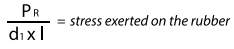In fact it also depends on the “enclosure” ratio l/D and the thickness of the elastic component s=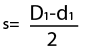The permissible stress (i.e. the maximum stress which the elastic components can bear) depends on the physical characteristics of the material, i.e. on its hardness measured in degrees according to the Shore A method.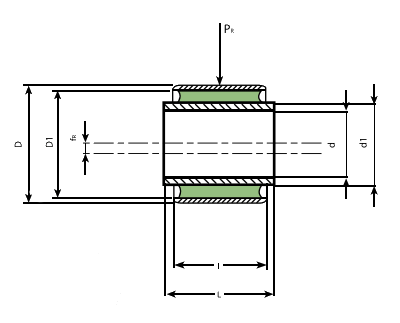### AXIAL CHARACTERISTICS

The applied force acts along the axis of the elastic joint. Consequent to the application of force PA an elastic axial deformation occurs measured by the axial displacement fA.

The maximum permissible axial static load can be calculated using the formula: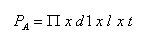t depends on the physical characteristics of the elastic component. In this case the joint is characterized by the maximum permissible static axial load PA and by the relevant displacement fA.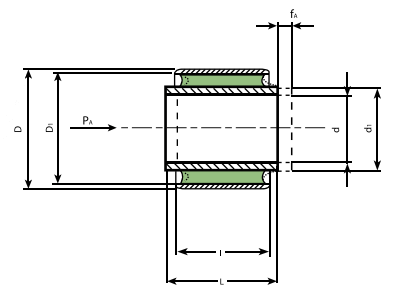### TORSIONAL CHARACTERISTICS

The application of a torque around the axis of the joint causes an angular deformation α.

Such deformation is counteracted by an elastic return torque. The maximum static permissible torque can be calculated using the formula: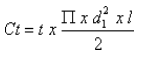t depends on the physical characteristics of the elastic component. The structure is characterized in this case by the maximum torque CT and the maximum permissible torsion angle α.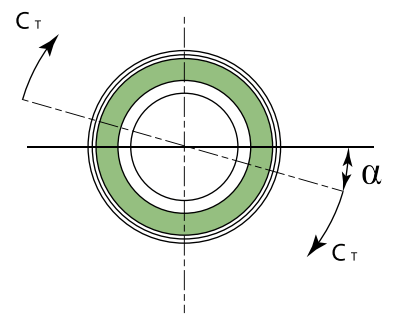### CONICAL CHARACTERISTICS

Applying a torque with the axis perpendicular to that of the joint causes an elastic deformation angle ß.

The angle ß has a maximum deformation permissible which corresponds to a torque of elastic return.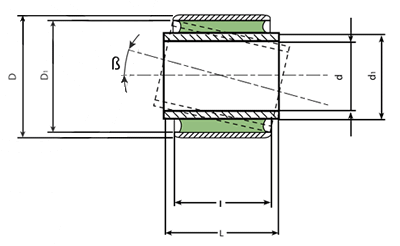## DYNAMIC CHARACTERISTICS

Where dynamic loads are involved, load values given in the tables of the catalog must be reduced according to the coefficient r.

In the case of loads of short duration and infrequent (shocks), loads can be doubled.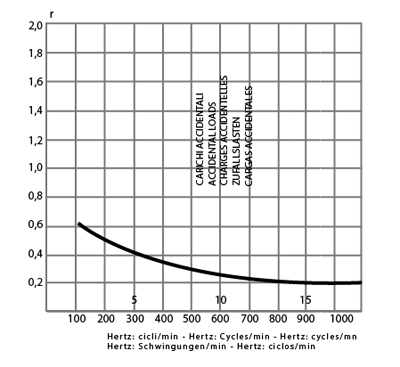### TORSIONAL AMPLITUDES

The torsional amplitudes indicated in the catalog must be reduced according to a coefficient t which depends on the frequency of the oscillation.### COMPOUND STRESS

Where more than one kind of load is simultaneously involved, the calculation of the stresses of the element becomes more complex. In this case, we recommend contacting our Technical Department.